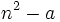# Every integer is a quadratic residue for infinitely many primes

## Statement

Letbe a nonzero integer. Then, there are infinitely many primessuch thatis a quadratic residue modulo. (The statement also holds for, if we declareto be a quadratic residue).

## Proof

By fact (1), there are infinitely many primessuch thatdividesfor some natural number. We can exclude the primes that divideitself (if we follow the convention thatis not considered a quadratic residue) and we still obtain that there are infinitely many primesfor whichis a quadratic residue.

Note that this proof generalizes to-adic residues for any.